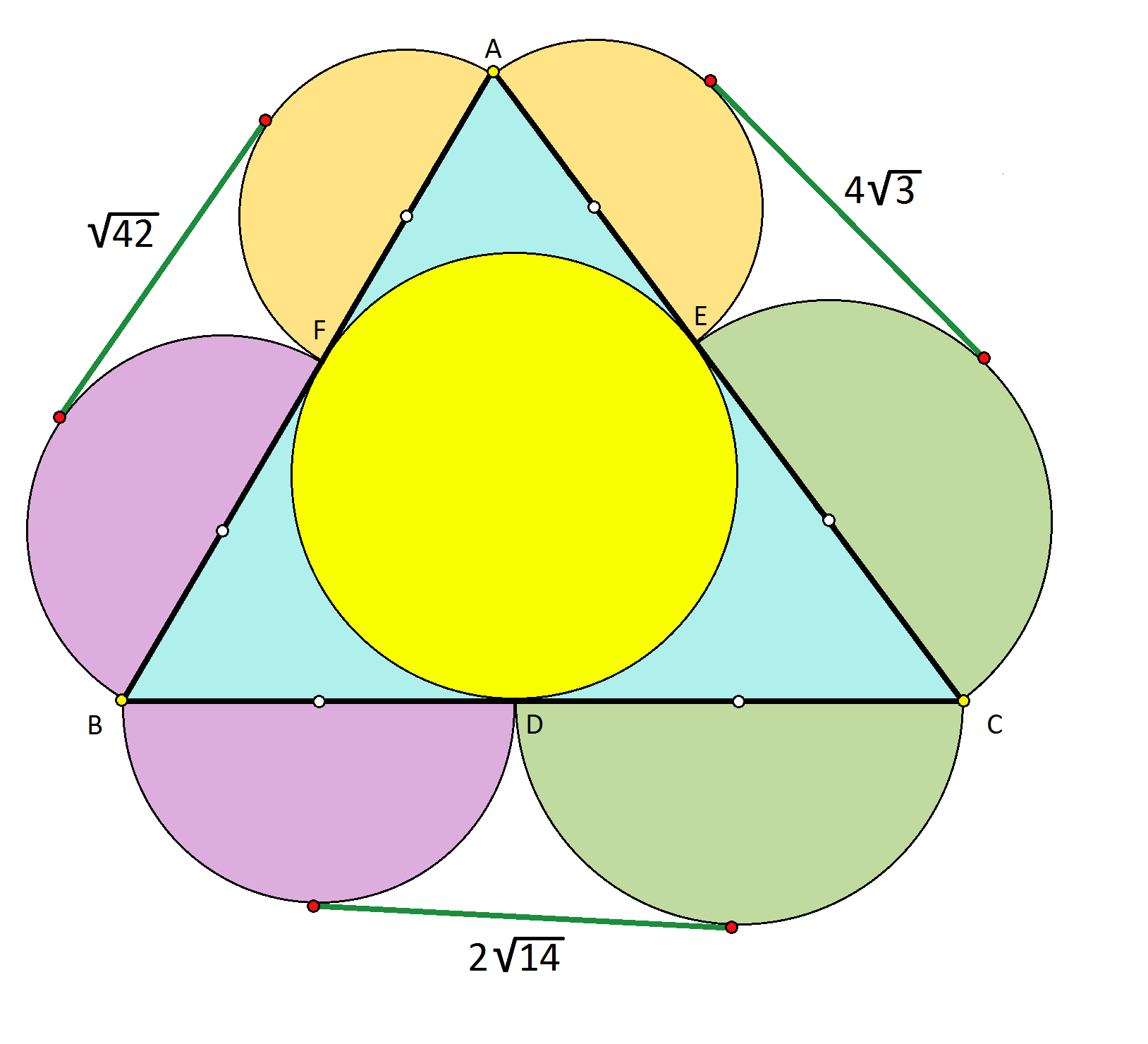# Classical geometry 2

Geometry Level 5In the figure to the right, the yellow circle is tangent to $\triangle ABC$ at points $D, E, F.$

$2 \sqrt{14}, 4 \sqrt{3},$ and $\sqrt{42}$ are the lengths of the common tangents to the semicircles of diameters $(BD, CD), (CE, AE),$ and $(AF, BF),$ respectively.

What is the radius of the yellow circle?

×